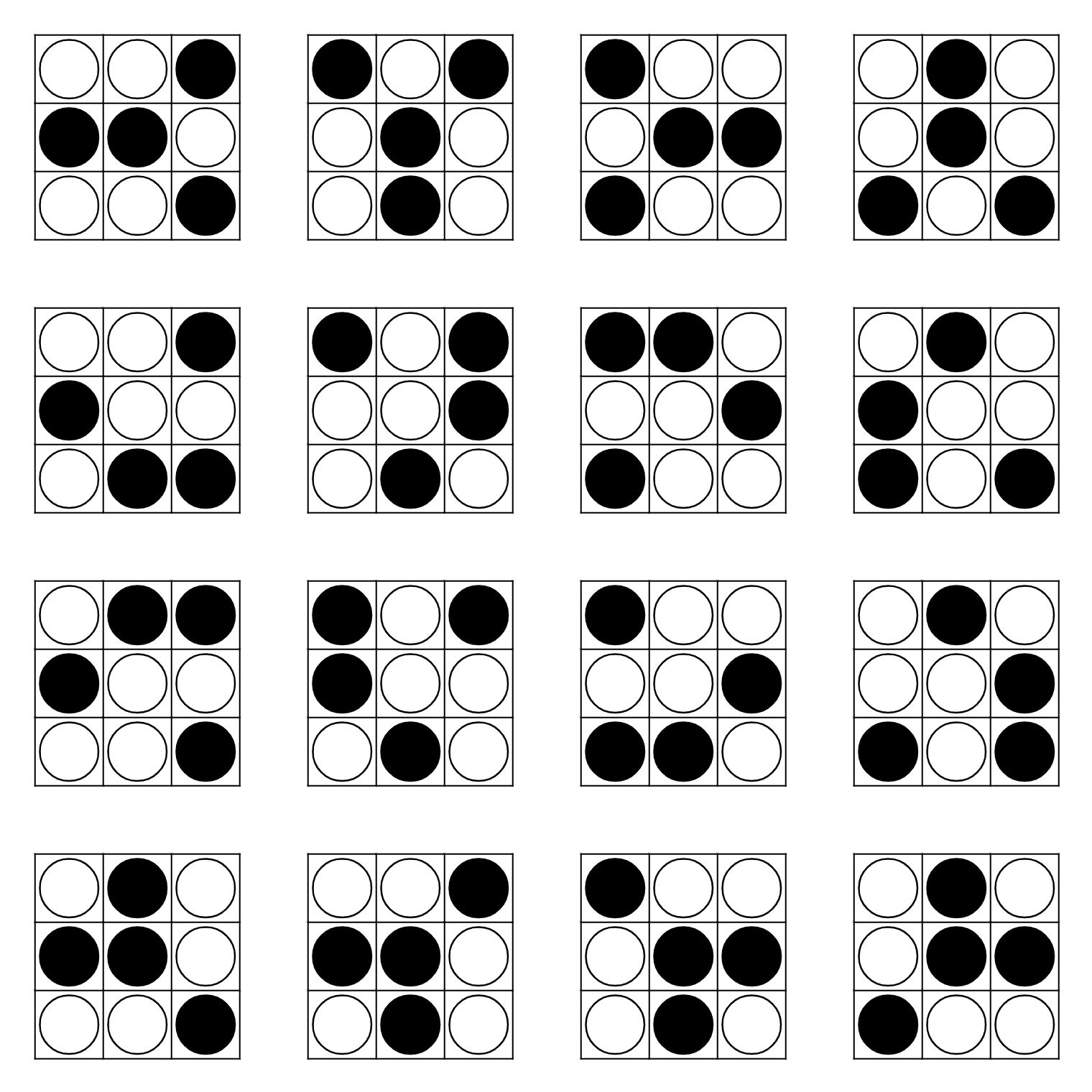# Counting Tic-Tac-Toe draws on larger grids

Alice and Bob play a game of Tic-Tac-Toe on a grid of size $$N \times M$$. The rules of this game are the same as the original Tic-Tac-Toe:

• Alice plays first (white); Bob plays second (black).
• On each player's turn, they put a piece of their own on an empty cell on the grid.
• When a player's pieces form a three-in-a-row (horizontally, vertically, or diagonally in either 45-degree direction), the player wins.
• If no one wins until the entire board is filled up with pieces, the game ends in a draw.

Instead of trying to win, Alice and Bob decided to cooperate towards a draw. How many distinct drawn endgames are possible when $$N, M \ge 4$$? Two endgames are distinct if the pieces on at least one cell is different. The order in which each piece is placed is not considered.

For example, $$3 \times 3$$ grid has 16 distinct drawn endgames:When one side of the grid is 3, the answer is given as OEIS A339631.

• Is there an elegant solution, or is this basically a computer programming assignment? Aug 7 at 5:48
• @KrisVanBael This can be solved without programming, unlike when $N=3$. Aug 7 at 5:52

18 if M=N=4, 14 if M=4 or N=4 but not both EDIT 8 if M,N>4 and their product is divisible by 4. 4 if M,N both odd and 6 otherwise.

Reasoning:

There can be no 2x2 square of one colour because any containing 3x3 would have to fill all other squares with the other colour which would then win.

    o o .             o o x
o o .   forces    o o x
. . .             x x x


2x2 squares with three pieces of one colour can only appear at the corners because

    o o .             o o x
o x .   forces    o x .
. . .             x . .

    o o . .             o o x .
x o . .   forces    x o o .
. . . .             . x x x

    x o . .             x o x o
o o . .   forces    o o x x
. . . .             x x o o
. . . .             o . . x


up to symmetry w.r.t. the diagonal. Such corner pieces can only happen on 4xN or Mx4 boards and can only occur in pairs.

If M,N>4 therefore every 2x2-square must be balanced and there are only 8 viable 3x3 squares namely the rotations and complement of

    o o x
x x o
o o x


As any sub 3x3 square determines all others (via unique 3x2 or 2x3 overlap with shifted-by-1 locations) 8 is also EDIT: an upper bound for the overall number of solutions. The solutions can be thought of as cuttings from rectangles made entirely from

    x o
x o
o x
o x


or its transpose. EDIT: Of those all that are overall balanced or have Alice one up are reachable.

If N=4<M there are 6 additional solutions depending on whether the left end is unbalanced or the right end or both each option coming with its complement. if M=N=4, there are another 4 (top two unbalanced or bottom two unbalanced plus complements)

• Your answer for N or M = 4 is correct, but the others is not. Hint: Alice and Bob take turns. (Sorry if it's not clear enough in the OP) Also I have to admit that the remaining part may be a bit tedious. Aug 7 at 9:20
• @Bubbler Oops. I hope it is fixed now. Aug 7 at 9:48